# STFT Spectrogram (Advanced Signal Processing Toolkit)

LabVIEW 2014 Advanced Signal Processing Toolkit Help

Edition Date: June 2014

Part Number: 372656C-01

»View Product InfoDownload Help (Windows Only)

The short-time Fourier transform (STFT) spectrogram is the normalized, squared magnitude of the STFT coefficients produced by the STFT. Normalization makes the STFT spectrogram obey Parseval's energy-conservation property, meaning that the energy in the STFT spectrogram equals the energy in the original time-domain signal. All the quadratic time-frequency analysis methods in the LabVIEW Time Frequency Analysis Tools adhere to Parseval's energy-conservation property.

The STFT spectrogram, a Cohen's class method, can be a good first choice for a quadratic time-frequency analysis method because this method is simple and fast. With the STFT spectrogram, you can infer if a signal is reasonably oversampled by looking for a low energy density at high frequencies in the STFT spectrogram. You also can estimate the spectral content of a signal and how the spectral content evolves over time by seeing where the energy is concentrated in the STFT spectrogram. However, other quadratic time-frequency analysis methods can provide superior time-frequency resolution. You can experiment with other methods in the Time Frequency Spectrogram Express VI to see if the STFT spectrogram unacceptably blurs the signal components you want to analyze.

When you have large data sets or when you do not need special features of the spectrogram, such as a fine time-frequency resolution, consider using the STFT spectrogram because it is fast and easy to use.

Use the TFA STFT Spectrogram VI to compute the STFT spectrogram.

## Window Type and Window Length

The STFT spectrogram usually is sufficient for most applications, but it typically provides a coarse time-frequency resolution as a result of window effects that the window type and the window length determine. A narrow window results in a fine time resolution but a coarse frequency resolution because narrow windows have a short time duration but a wide bandwidth. A wide window results in a fine frequency resolution but a coarse time resolution because wide windows have a long time duration but a narrow frequency bandwidth. You cannot obtain a fine time resolution and a fine frequency resolution simultaneously by using the STFT spectrogram.

### Selecting an Appropriate STFT Spectrogram Window Length

The following figure shows a frequency hopper signal, commonly used in spread-spectrum communication systems, such as CDMA cell phones.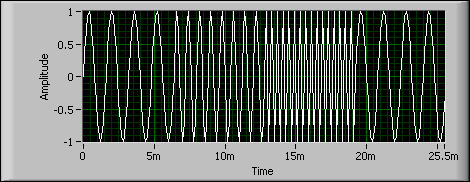The following figure shows the ideal quadratic time-frequency representation of the example frequency hopper signal in the previous figure.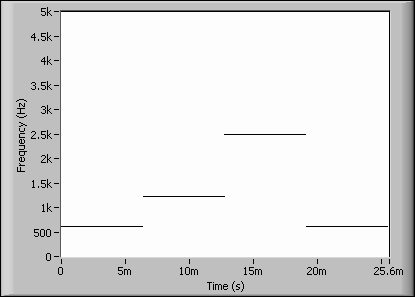In the previous figure, the ideal representation of the frequency of the signal remains constant and then immediately switches to another frequency. The following figure shows the STFT spectrogram of the example frequency hopper signal with a window length of 128.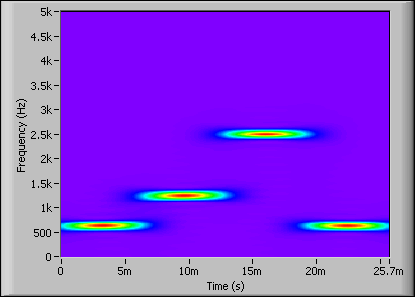Compared to the ideal time-frequency representation, the energy distribution of the signal in the previous figure is not confined to narrow lines. The STFT spectrogram in the previous figure is blurry as a result of the window effects of the STFT. This blurriness is a manifestation of the coarse time-frequency resolution of the STFT spectrogram. The window length and the window type determine how the energy blurs across time and frequency and thus determine the time resolution and the frequency resolution of the STFT spectrogram. For example, a wider window length can reduce energy blurring across frequencies at the expense of increased blurring across time. A narrower window length can reduce blurring across time at the expense of increased blurring across frequencies. The following figure shows the STFT spectrogram of the example frequency hopper signal with a window length of 32.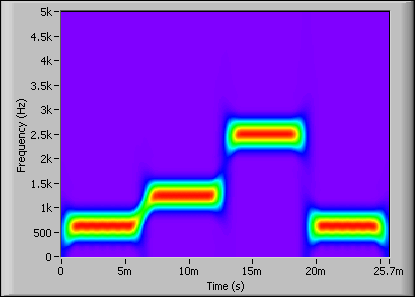Relative to the STFT spectrogram of the frequency hopper signal with a window length of 128, the energy distribution of the signal in the previous figure is more spread out along the frequency axis and is more compact along the time axis, which means that the STFT spectrogram has a coarser frequency resolution but a finer time resolution when the window length is narrow.

The following figure shows the STFT spectrogram of the example frequency hopper signal with a window length of 256.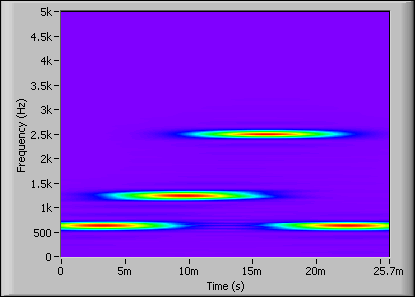Relative to the spectrogram of the frequency hopper signal with a window length of 128, the energy distribution of the signal in the previous figure is more spread out along the time axis and is more compact along the frequency axis, which means that the STFT spectrogram has a coarser time resolution but a finer frequency resolution when the window length is wide.

Refer to the Time-Frequency Resolution VI in the labview\examples\Time Frequency Analysis\TFAGettingStarted directory for an example that demonstrates the window effects of the STFT spectrogram.

## Reassignment Method

You can use the reassignment method to improve the time-frequency resolution of the STFT spectrogram artificially. The reassignment method automatically compresses the energy in the quadratic time-frequency representation toward the centers of gravity of the signal components to make the signal components more concentrated. The reassignment method uses the assumption that the energy of the signal components in the time-frequency representation is tightly concentrated. Using the reassignment method can help improve the time-frequency resolution of time-frequency concentrated components. However, the reassignment method also can bias the location of spectral peaks, merge distinct spectral components, falsely split compact signal components, or excessively sharpen naturally blurry signal components in the resulting time-frequency representation. The following figure shows the reassigned STFT spectrogram of the example frequency hopper signal.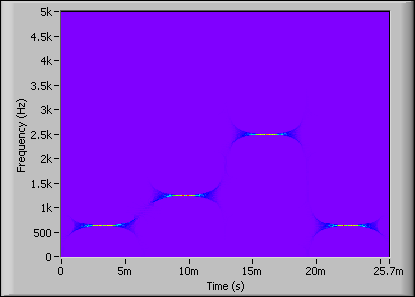Notice that the signal components in the reassigned STFT spectrogram in the previous figure are less blurry in time and frequency than the STFT spectrograms in the figures of the frequency hopper signal with a window length of 128, 32, and 256. Thus, this reassigned STFT spectrogram has a better time-frequency resolution.